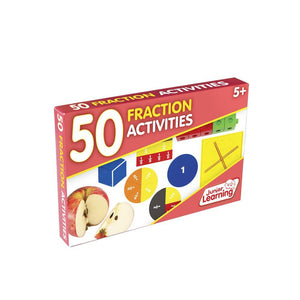# 50 Fraction Activities

Item # JL331
• \$14.99

50 Fraction Activities is a set of 50 double-sided activity cards for teaching fraction knowledge and understanding. Topics include: equivalent fractions, comparing fractions, fractions of a set, simple division, adding fractions, subtracting fractions, simplifying fractions, improper fractions, multiplying fractions, dividing fractions, common denominator, mixed numerals and decimal fractions. The front side provides teaching objectives, resources used and procedure. The other side provides visual examples. Math manipulatives used effectively throughout and presented in clear photography. Contains 50 double-sided activity cards and a contents card that can act as a check off list.